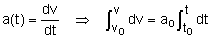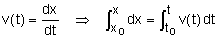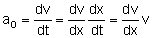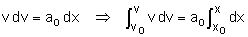Ch 1. Particle General Motion Multimedia Engineering Dynamics Position,Vel & Accel. Accel. varyw/ Time Accel. Constant Rect. Coordinates Norm/Tang. Coordinates Polar Coordinates RelativeMotion
 Chapter - Particle - 1. General Motion 2. Force & Accel. 3. Energy 4. Momentum - Rigid Body - 5. General Motion 6. Force & Accel. 7. Energy 8. Momentum 9. 3-D Motion 10. Vibrations Appendix Basic Math Units Basic Equations Sections Search eBooks Dynamics Fluids Math Mechanics Statics Thermodynamics Author(s): Kurt Gramoll ©Kurt GramollDYNAMICS - THEORY

Many times, the motion of an object is specified by an acceleration that is constant with time. For example, an object that falls for a short distance in the earth's (or any other planet's) atmosphere experiences a constant acceleration. That constant is written as, ao,

a = dv/dt = ao = constant

By integrating, the velocity can be determined as a function of the acceleration and time, givingv(t) = vo + ao (t - to)

Next, the velocity can be integrated to express the position as a function of the acceleration and time, giving,x(t) = xo + vo (t - to) + ao (t - to)2 / 2

Using the chain rule, the acceleration can be expressed asThis relationship can be integrated to express the velocity as a function of the acceleration and position.v2 = vo2 + 2 ao (x - xo)

It should be stressed that above equations are only valid when the acceleration is expressed as a constant.

Practice Homework and Test problems now available in the 'Eng Dynamics' mobile app
Includes over 400 problems with complete detailed solutions.
Available now at the Google Play Store and Apple App Store.